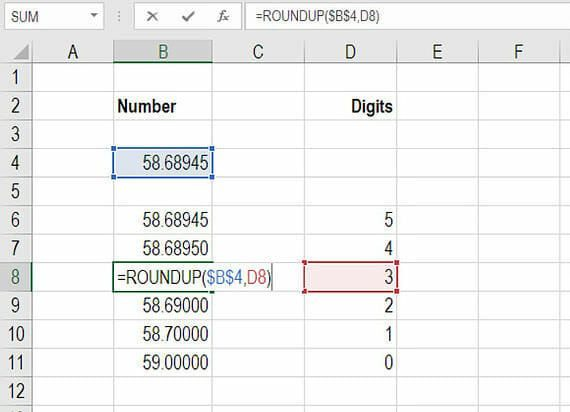ROUNDUP Excel

How to round a number up in Excel.

How to use Excel ROUNDUP

The Excel ROUNDUP function allows a user to round a number up in Excel, to a certain number of decimal points. For anyone who performs financial analysis in Excel it can frequently be important to round a number to a specific decimal place or to a whole number.

In this session, we will show you how to round a number up in Excel using the =ROUNDUP() function.

This formula will be a major help in saving you time when performing financial modeling and will also improve your accuracy in Excel.

How the Excel ROUNDUP function works

The Excel roundup formula asks you to reference a specific number and then indicate how many units (decimal places) you want to round it to.

=ROUNDUP()

In this example, we show you how to create a table that uses the roundup formula to link to a number and then return a certain number of decimal places.

Step 1: type “=ROUNDUP(“

Step 2: link to the cell you want to round and add a comma

Step 3: type the number of units, or link to a cell that contains the number of units you want to display

Step 4: close bracket and press enter.

See the screenshot below for an example of the “roundup” function in action.As you can see in the table above, the number 58.68945 can be rounded to anywhere from 0 to 5 decimal pages.  In order to use the function, simply refer to cell B4 and any of cells D6 to D11 to specify the number of decimal places to include.

More CFI Excel resources

Thank you for reading CFI’s guide to the Excel ROUNDUP function. If you want to become an Excel power user please check out our Excel Resources to learn all the most important functions, formulas, shortcuts, tips, and tricks.

• Excel Round Down
• Insert today’s date
• Waterfall chart in Excel
• INDEX MATCH
• Excel for Dummies

Free Excel Tutorial

To master the art of Excel, check out CFI’s FREE Excel Crash Course, which teaches you how to become an Excel power user.  Learn the most important formulas, functions, and shortcuts to become confident in your financial analysis.

Launch CFI’s Free Excel Course now to take your career to the next level and move up the ladder!